## Sunday, January 27, 2013

### pystruct: more structured prediction with python

Some time ago I wrote about a structured learning project I have been working on for some time, called pystruct.
After not working on it for some time, I think it has come quite a long way the last couple of weeks as I picked up work on structured SVMs again. So here is a quick update on what you can do with it.

To the best of my knowledge this is the only tool with ready-to-use functionality to learn structural SVMs (or max-margin CRFs) on loopy graphs - even though this is pretty standard in the (computer vision) literature.

The most commonly used software for learning structural SVMs is SVM^struct by Thorsten Joachims, which is a great piece of software, but imho not that easy to use and written completely in C (with a Python interface, though).

A quick reminder on what structured prediction does (I wrote about this before). So you are given a list of input objects $X_1, ..., X_n$ and corresponding $Y_1, ..., Y_n$, and you want to learn to predict the output $Y$ for some unknown input $X$.

This is a generalization of standard a multi-class classification in two ways:
•  The input objects $X$ are structured, meaning they are not just an array of numbers, as usually the case in machine learning, but something more complex, for example a sequence or an image or a graph. There are usually some numerical values associated with X, but it is somehow more than just a flat vector.
• The output objects $Y$ usually belong to some very large set. Think of labelling a sequence of length $r$, where each entry can take one of $m$ classes. Then the set of possible $Y$ has size $m^r$ - which grows exponentially fast in the lenght of the sequence. To cope with this, $Y$ is also assumed to have some structure, that can help us generalize in the presence of so many classes.

The mathematical formulation of structured prediction is
$Y = f(X) = \text{argmax}_{\hat{Y}} g(X, \hat{Y}, \theta)$

Here $g$ is a function that encodes the compatibility of $\hat{Y}$ with $X$ and depends on a set of parameters $\theta$. The prediction $Y$ is given as that $\hat{Y}$ that maximizes the compatibility with $X$.

If you want to view this in a probabilistic way, you can replace $g(X, \hat{Y}, \theta)$ by $p(\hat{Y}| X, \theta)$. Then the prediction $Y$ is the maximum a postiory (MAP) estimate for $Y$.

The task of structured prediction is to find parameters $\theta$ such that we predict well on future data $X$.

There are several steps in doing this. First, we have to say what "good" means.
A common measure is the hamming loss on $Y$. So for the sequence example above, we don't punish all possible outputs equally, but penalize based on how many entries of a predicted sequence are wrong.

As we can't know about future data, we do the standard thing of regularized empirical risk minimization. In English, this means we want a model that does well on the training data and is simple. Usually this is formulated as

$\min_\theta L(\mathbf{X}, \mathbf{Y}, \theta) = \sum_{i=1}^n l(f(X_i), Y_i) + \frac{1}{C} R(\theta)$
where $l$ is for example the Hamming loss and $R$ is some penalty on the complexity of the parameters, for example the euclidean (L2) length and $C$ is a trade off between complexity and goodness of fit.

The next step is choosing the form of $g$. In pystruct, I implemented the structural SVM and structural Perceptron approaches, which use a simple linear form:
$g(X, \hat{Y}) = \left<\theta, \psi(X, \hat{Y}) \right>$

Here, $\psi(X, \hat{Y}) \in \mathbb{R}^d$ is a joint feature of $X$ and $\hat{Y}$ and $\theta$ is simply a vector of weights. All the structure of the problem is encoded in $\psi$.

Now that we have all the math together, we can see there are several parts to the problem:

1. Defining and computing $\psi$, the joint feature of $X$ and $\hat{Y}$ that defines the structure of the problem.
2. Computing $\text{argmax}_{\hat{Y}} g(X, \hat{Y}, \theta)$.
3. Find the best parameter settings $\theta$ by solving
$\min_\theta L(\mathbf{X}, \mathbf{Y}, \theta),$
i.e. the actual learning part.
There are three modules in pystruct, corresponding to these there parts.
(For reference, SVM^struct concentrates on solving 3. - and does a great job at it).

#### 1. Problems (i.e. CRFs): Knows about the problem structure.

These know about the structure of the problem, the loss and the inference.
This is basically the part that you have to write yourself when using the
Python interface in SVM^struct.
I am only working on pairwise models and there is support for grids and
general graphs. I am mostly working on the grids at the moment.

#### 2. Inference Solvers: Does the heavy lifting in inference.

There are some options to use different solvers for inference.
A linear programming solver using GLPK is included.
I have Python interfaces for several other methods on github,
including LibDAI, QPBO, AD3, which can all be easily used with pystruct.

This is where the heavy lifting is done and in some sense these backends
are exchangeable.

#### 3. Learners: Know about learning.

These implement max margin learning, similar to SVM^struct.
There is an online subgradient version, a one-slack QP version and the
standard n-slack QP version. The QPs are solved via cvxopt.

They are not particularly optimized but getting there.
Often this is not the bottleneck when working with loopy graphs.
There is also a simple perceptron. I might be adding an interface to SVM^struct here, if I'm not happy with my current solvers in the future.

Now let the code speak. First, let us look at some ways to use the library.
I definitely want to explain the inner workings, too, but that might be another post.
pystruct includes several examples. Lets walk through a simplified version of the binary svm example first, and work our way up from there.

The example simply shows how to implement a standard binary SVM in the pystruct framework. This is just an illustration - don't use that to actually solve your SVM problems, as it is not really optimized for that ;)

### Binary SVM

First, we load some data using scikit-learn. We use the digits dataset and make it into a binary prediction task. We add a column of ones as the structural SVM doesn't implement a bias term.
In :
import numpy as np

from sklearn.datasets import load_digits
from sklearn.cross_validation import train_test_split

# do a binary digit classification
X, y = digits.data, digits.target

# make binary task by doing odd vs even numers
y = y % 2
# code as +1 and -1
y = 2 * y - 1
X /= X.max()

X_train, X_test, y_train, y_test = train_test_split(X, y)
X_train_bias = np.hstack([X_train, np.ones((X_train.shape, 1))])
X_test_bias = np.hstack([X_test, np.ones((X_test.shape, 1))])

The data here is actually not structured, just an array.
In :
X_train_bias.shape

Out:
(1347, 65)
The labels are also not structured:
In :
y_train.shape

Out:
(1347,)
The two labels are encoded as -1 and +1 as common in SVMs.
In :
y_train

Out:
array([ 1, -1, -1, ..., -1,  1,  1])
Now we first import and instantiate the problem description for a binary SVM.
In :
from pystruct.problems import BinarySVMProblem
problem = BinarySVMProblem(n_features=X_train_bias.shape)

Now we import one of the learners. These are completely agnostic to the kind of problem we want to solve. Let's try the online subgradient method. We provide it with the instance of the binary SVM formulation, set the regularization parameter $C$ in the terminology from above)
In :
from pystruct.learners import SubgradientStructuredSVM
ssvm = SubgradientStructuredSVM(problem, C=20, learning_rate=0.0001, max_iter=50)

Now we train the algorithm. The learners all have the usual scikit-learn interface:
In :
ssvm.fit(X_train_bias, y_train)

Training primal subgradient structural SVM
final objective: 0.339834
calls to inference: 67350

And evaluate on the test set:
In :
ssvm.score(X_test_bias, y_test)

Out:
0.88
Let's have a look at some predictions:
In :
np.array(ssvm.predict(X_test_bias))[:5]

Out:
array([-1.,  1.,  1.,  1., -1.])
That wasn't very hard now. But also not terribly exciting.
So, a little step up in complexity:

### Multi-Class SVM.

The Crammer-Singer multi-class formulation is a special case of a structural SVM.
Let's get the original labels back:
In :
y = digits.target
X_train, X_test, y_train, y_test = train_test_split(X, y)
X_train_bias = np.hstack([X_train, np.ones((X_train.shape, 1))])
X_test_bias = np.hstack([X_test, np.ones((X_test.shape, 1))])

In :
y_train

Out:
array([8, 5, 2, ..., 4, 9, 3])
In :
from pystruct.problems import CrammerSingerSVMProblem

In :
problem = CrammerSingerSVMProblem(n_features=X_train_bias.shape, n_classes=10)

In :
ssvm = SubgradientStructuredSVM(problem, C=20, learning_rate=0.0001, max_iter=50)

In :
ssvm.fit(X_train_bias, y_train)

Training primal subgradient structural SVM
final objective: 0.619628
calls to inference: 67350

In :
ssvm.score(X_test_bias, y_test)

Out:
0.92000000000000004
In :
np.array(ssvm.predict(X_test_bias))[:5]

Out:
array([5, 5, 2, 0, 2])
But no let us finally get to something a bit more interesting

### CRFs on grid graphs

Actually pystruct contains some code to handle grid-graphs but to demonstrate the interface, I'll represent the graphs explicitly. First, let us generate some 2d grid toy data.
In :
import pystruct.toy_datasets as toy
from pystruct.utils import make_grid_edges

X, Y = toy.generate_blocks(n_samples=3)

We generated 3 examples. Each example here is a 10 x 12 grid with two possible labels, 0 and 1:
In :
print(Y.shape)
plt.matshow(Y[0, :, :])

(3, 10, 12)

Out:
<matplotlib.image.AxesImage at 0x67bf650>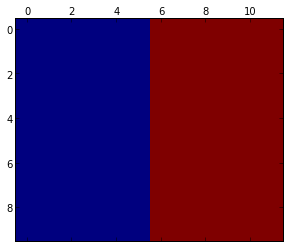The input is a noisy version of this label (actually there are two features per point in the grid).
In :
print(X.shape)
plt.matshow(X[0, :, :, 1])

(3, 10, 12, 2)

Out:
<matplotlib.image.AxesImage at 0x69c2910>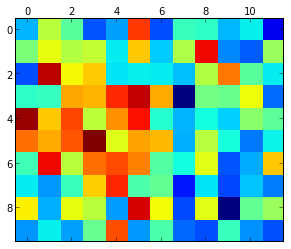We hope that the pairwise CRF can learn to smooth the data to get consistent, sharp labels out.
Now we import the class for handling CRFs with arbitrary (gobally shared) pairwise potentials, GraphCRF and instantiate it. As for such a grid graph the inference is already non-trivial, we have to choose an inference method. Let's go with AD3 for the moment.
In [ ]:
crf = GraphCRF(inference_method='ad3')
clf = SubgradientStructuredSVM(problem=crf, max_iter=200, C=100)

To feed the instances to pystruct, we explictly represent each edge in the grid:
In [ ]:
G = [make_grid_edges(x) for x in X]

In :
G[:12]

Out:
array([[ 0,  1],
[ 1,  2],
[ 2,  3],
[ 3,  4],
[ 4,  5],
[ 5,  6],
[ 6,  7],
[ 7,  8],
[ 8,  9],
[ 9, 10],
[10, 11],
[12, 13]])
Now we reshape the input a bit. GraphCRF expects the input X to be of shape (n_nodes, n_features) and the output Y to be of shape (n_nodes,) for each sample.
In [ ]:
# reshape / flatten x and y
X_flat = [x.reshape(-1, 2) for x in X]
Y_flat = [y.ravel() for y in Y]

The actuall input when using GraphCRF is then a tuple for each sample x=(features, graph).
In [ ]:
X_structured = zip(X_flat, G)

Then the rest is easy again:
In [ ]:
clf.fit(X_structured, Y_flat)
Y_pred = clf.predict(X_structured)

Now let us look at the prediction:
In :
plt.matshow(Y_pred.reshape(Y.shape))

Out:
<matplotlib.image.AxesImage at 0x6bc7b50>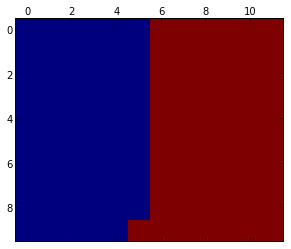In [ ]:
And compare against just using the features, without using any interaction between the nodes:

In :
plt.matshow(X[0, :, :, 1] > 0.0)

Out:
<matplotlib.image.AxesImage at 0x7379390>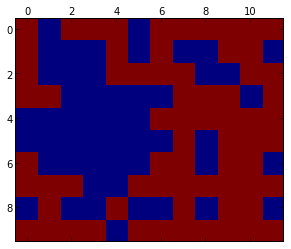I'm getting a bit tired. Expect the post to be updated around tomorrow ;)

1.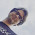Andy, it seens that in line 31 of examples/binary_svm.py SubgradientStructuredSVM has no parameter 'batch'.

Nice Work ;)

1.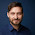Thanks. Should be fixed now :)

2.This comment has been removed by a blog administrator.

3.hi..Im college student, thanks for sharing :)

4.This comment has been removed by the author.